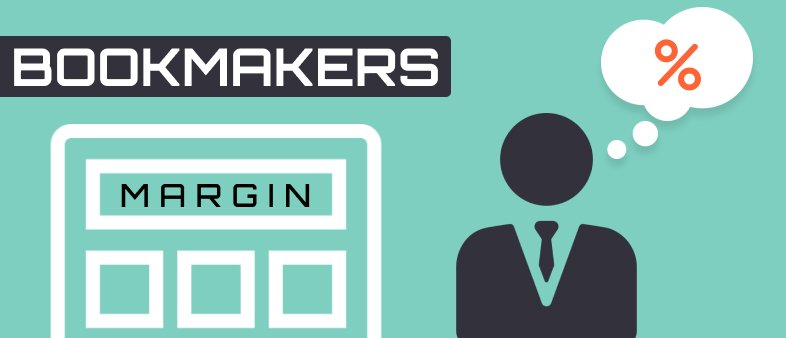Betting school

# Bookmakers margin: What is it and how to calculate it

The bookmakers’ margin is the amount of profit as a % that they should make on average on each game. The size of the margin directly affects the value of the odds they offer, the lower it is, the more profitable the odds are for the punters.The bookmakers do not usually advertise the size of the margins, and they can be different from game to game. To find out what the margin is often you need to calculate it yourself.

To demonstrate exactly what we mean by the margin it is best explained with just an ordinary coin. As we know, the probability of it landing on heads or tails is the same, there is a 50% chance for each. And if the bookmaker took bets on this event, if there was no margin then they would give the same odds of Evens (decimal 2) on each.

You bet on the heads, your friend on tails, and in either outcome all the money delivered will be returned to one of the players since someone will win. However, if you do this then the bookmaker will never make a profit.  Therefore, to operate as a business the bookmaker will instead allow punters to bet on 50/50 events but not with odds of 2 but instead say 1.91.  Now with two opposite bets of \$100, each \$91 profit, and the bookmaker would make \$9 profit every time regardless of the result.

In the context of this example, in the long run, it would be impossible to profit, but in other events where you show a predictive talent for making bets, and it is not obvious what the true odds should be, then the margin becomes just an additional barrier.

At a margin of 4 percent, you have to be able to analyse 4 percent more accurately than the bookmaker what the true odds are, in order to profit. The height of this barrier (size of the margin) varies depending on the bookmaker, the popularity of the event you are betting on, the sport and also between individual lines on the game. It is most convenient to calculate the margin as a percentage.

## How to calculate a margin as a percentage?

It is most frequent to have to calculate margins with events which have two or three options to choose from.

The calculation formula for outcomes with two options:

Margin = 1 - 1 / (Probability 1 + Probability 2), where Probability 1 = 1 / Ratio 1, and Probability 2 = 1 / Ratio 2.

For example, let's take one of the most popular sports for betting; Tennis. In the final of the US Open 2018, Novak Djokovic and Juan Martin del Potro met. The bookmaker took bets on the victory of Djokovic with odds of 1.45 and on del Potro at odds of 2.84.

Margin = 1 - 1 / (1 / 1.45 + 1 / 2.84) = 4%.

The calculation formula for bets with three options:

Margin = 1 - 1 / (Probability 1 + Probability 2 + Probability 3), where Probability 1 = 1 / Ratio 1, Probability 2 = 1 / Ratio 2, Probability 3 = 1 / Ratio 3.

Consider the 2020 Champions League football match between "Liverpool" and "Atletico Madrid". The bookmaker rated Atletico's victory with odds of 7.15, a draw with 4.05 and Liverpool to win at odds of 1.58. We calculated the margin based on the proposed odds:

Margin = 1 - 1 / (1 / 7.15 + 1 / 4.05 + 1 / 1.58) = 1.93%.

The Champions League is one of the leading tournaments in the world in terms of betting volumes, so the competition leads to bookmakers having a very low margin.

## Tips for beginners about betting margins

From the examples presented, it may seem that the margin in football is lower than in tennis, but that is not always true.  It will depend on each individual bookmaker how they prioritise their odds and each bookmaker will have its own tactics to attract players. The size of the margin is just one of these tools.

When choosing a bookmaker, you should always be trying to find main outcomes with a 3% margin and in maybe in some minor betting lines or less mainstream matches, (say, in the number of corners in a League 2 game), you will face a margin instead of nearer 7%.

All bookmakers margins will vary from event to event and the advantageous odds for the Champions League are not a guarantee that the same bookmaker will make a generous offer for baseball or cricket. Understanding margins though and using the formula to calculate them, can really help you find a bookmaker which is offering value.

Note! The main thing to remember is that the margin is important, sure, but it is just one of the many factors you need to consider. For example, in the vegetable market you buy not only from the seller with the lowest price, but you also consider where the products were grown, are they fresh, are they organic, or even just is the seller pleasant to talk to as a person.

When choosing a bookmaker, you consider a combination of factors, because you need to play responsibly and have a good time while doing it.